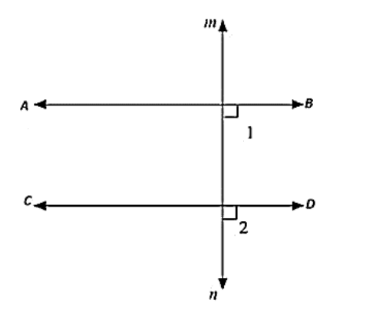# Prove that the straight lines perpendicular to the same straight line are parallel to one another.

Question:

Prove that the straight lines perpendicular to the same straight line are parallel to one another.Solution:

Let AB and CD be drawn perpendicular to the Line MN

∠ABD = 90°   [AB is perpendicular to MN]  .... (i)

∠CON = 90°   [CD is perpendicular to MN ]  .... (ii)

Now,

∠ABD = ∠CDN = 90° [From (i) and (ii)]

Therefore, AB ∥CD, Since corresponding angles are equal.